Courses

# Complex Functions Civil Engineering (CE) Notes | EduRev

## Civil Engineering (CE) : Complex Functions Civil Engineering (CE) Notes | EduRev

The document Complex Functions Civil Engineering (CE) Notes | EduRev is a part of the Civil Engineering (CE) Course Topic wise GATE Past Year Papers for Civil Engineering.
All you need of Civil Engineering (CE) at this link: Civil Engineering (CE)

Q. 1 Given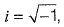the value of the definite integral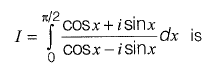[2015 : 1 Mark, Set-II]

(a) 1
(b) -1
(c) i
(d) - i

Ans: c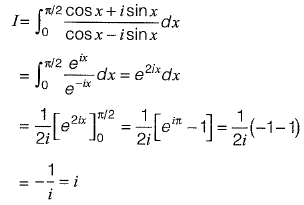Q. 2 Consider the following complex function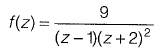Which of the following is one of the residues of the above function ?
[2015 : 2 Marks, Set- 1]

(a) -1
(b) 9/16
(c) 2
(d) 9
Ans: a

f(z) has poles at z = 1, - 2 Residue of f(z ) at ( z = 1)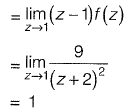Residue of f(z) at ( z = -2 )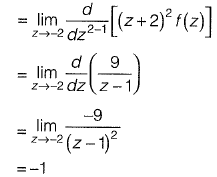Q. 3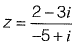can be expressed as
(a) - 0.5 - 0.5i
(b) - 0.5 + 0.5i
(c) 0.5 - 0.5i
(d) 0.5 + 0.5i       [2014 : 1 Mark, Set-II]
Ans: (b)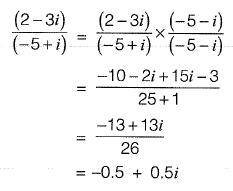Q. 4 For an analytic function, f{x; + iy) = u(x, y) + iv[x, y), u is given by u = 3x2- 3y2 . The expression for v, considering K to be a constant is
(a) 3 y2 -  3x2 + K
(b) 6x - 6 y + K
(c) 6y - 6x + K
(d) 6xy + K        [2011 : 2 Marks]

Ans (d)
f= u + iv
u = 3x2 - 3y2
For f to be analysis, we have Cauchy-Riemann conditions,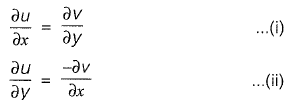From (i) we have,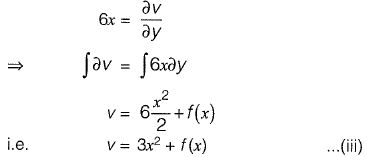Now applying equation (ii) we get,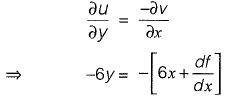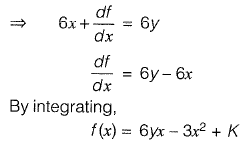Substitute in equation (iii),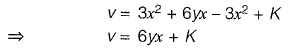Offer running on EduRev: Apply code STAYHOME200 to get INR 200 off on our premium plan EduRev Infinity!

69 docs

,

,

,

,

,

,

,

,

,

,

,

,

,

,

,

,

,

,

,

,

,

;